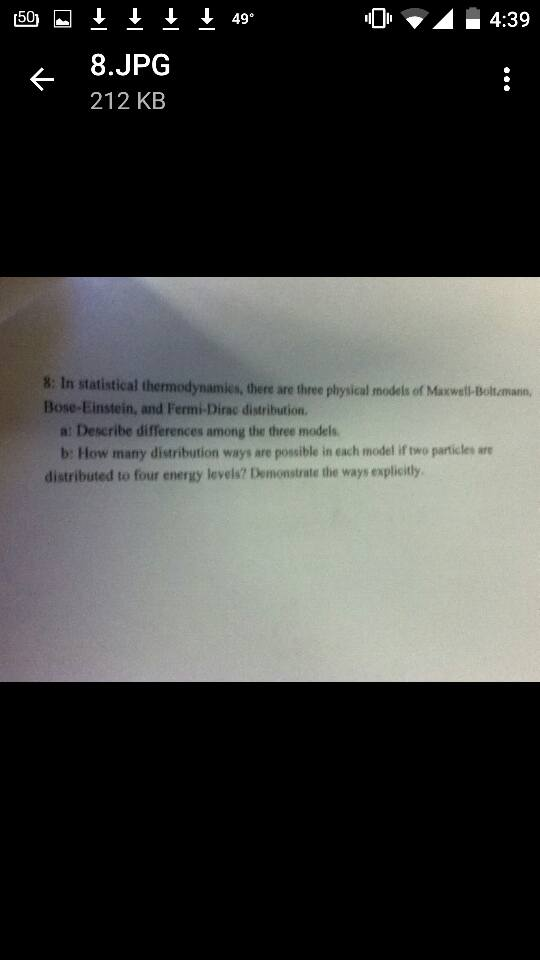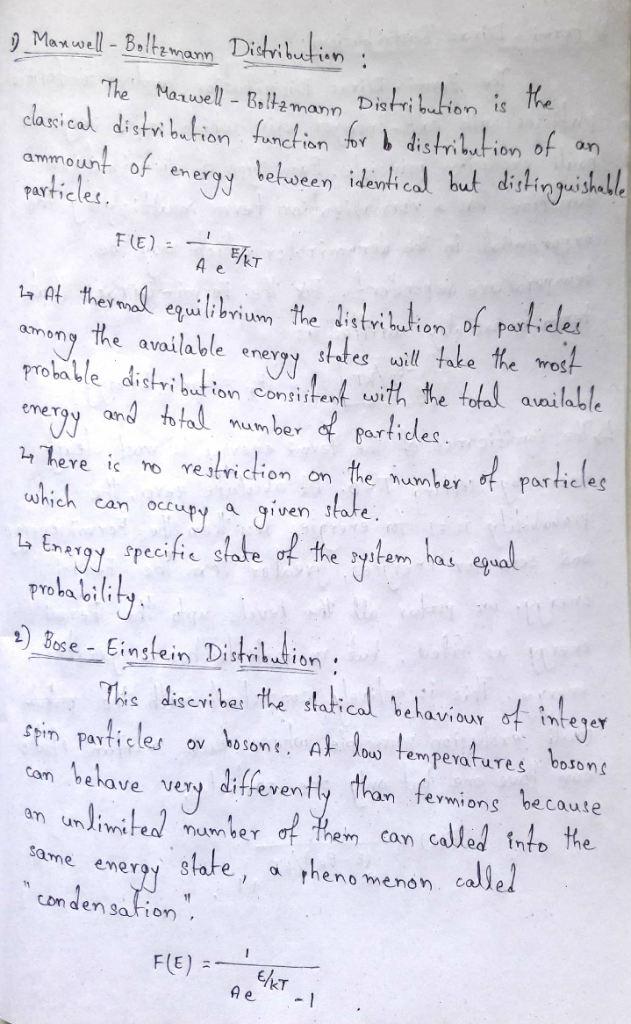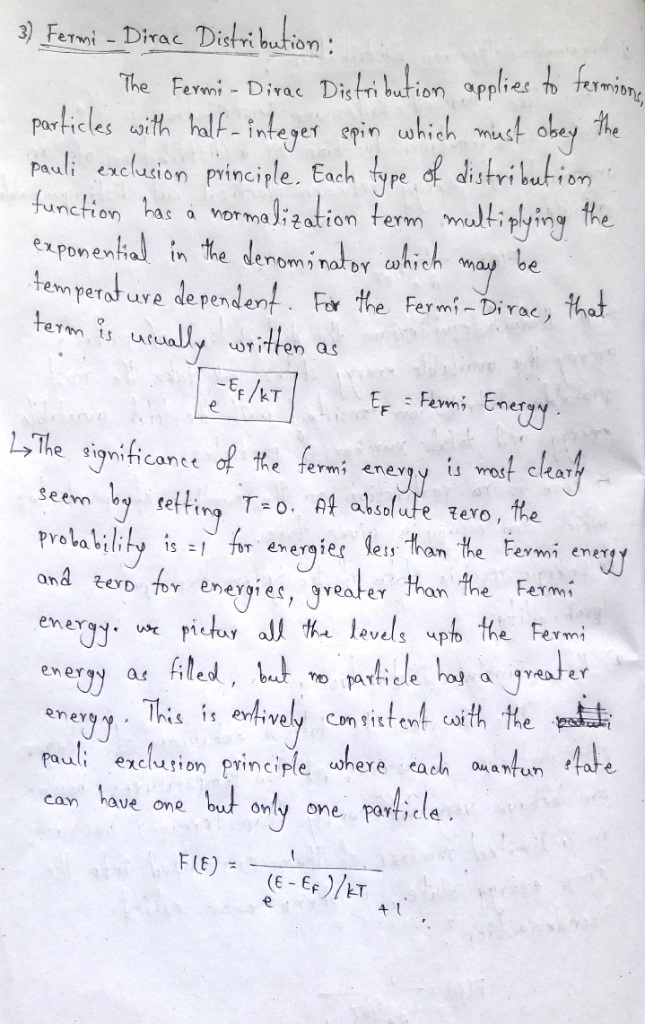# 4:39 49° 8.JPG 212 KB 8 In statistical thermodynamies, there are three physical models of Maxwell-Bolt.mann Bose-Einstein, and Fermi-Dirac distribution. a: Describe differences among the three models...4:39 49° 8.JPG 212 KB 8 In statistical thermodynamies, there are three physical models of Maxwell-Bolt.mann Bose-Einstein, and Fermi-Dirac distribution. a: Describe differences among the three models b: How many distribution ways are possible in each modet if two particles are distributed to four energy levels? Demonstrate the ways explicitly##### Add Answer of: 4:39 49° 8.JPG 212 KB 8 In statistical thermodynamies, there are three physical models of Maxwell-Bolt.mann Bose-Einstein, and Fermi-Dirac distribution. a: Describe differences among the three models...
More Homework Help Questions Additional questions in this topic.